Select Page

# Graphs

Data Structures Graphs

Contents

Define and classify the Graph 1

Recall various Terminologies used in the Graph 2

Describe various representations of a Graph 4

Compare various representations of a Graph 5

Define Graph Traversing 7

Describe BFS with Suitable example 8

Describe DFS with suitable examples 9

Write an Algorithm or a C Function for BFS and DFS 10

Describe Topological Sort of a Graph 15

Define Minimum Spanning Tree 17

Describe Kruskal’s Algorithm 18

Find Minimum Spanning Tree for the given Graph using Kruskal’s Algorithm 19

Describe Prim’s Algorithm 23

Find Minimal Spanning Tree for a given Graph using Prim’s Algorithm 24

Define the following terms: Transitive Closure, Activity Network, and Shortest Path 27

Discuss Warshall’s Algorithm 27

Find All Pair Shortest Paths for a given Graph using Warshall’s Algorithm 31

Describe Dijkstra’s Algorithm 33

Find the Single Source Shortest Path for the given Graph Dijkstra’s Algorithm 37

# Define and classify the Graph

In data structures, a graph is a non-linear data structure that consists of a set of vertices (also known as nodes) connected by edges. Graphs are used to represent relationships or connections between different elements. They are fundamental in solving problems related to networks, routing, scheduling, and optimization.

A graph G is typically defined as G = (V, E), where:

• V represents the set of vertices (nodes) in the graph.
• E represents the set of edges, which are the connections between vertices.

Classification of Graphs in Data Structures:

1. Directed vs. Undirected Graphs:
• In an undirected graph, the edges have no specific direction. The connection between two vertices is bi-directional.
• In a directed graph, the edges have a specific direction. The connection between vertices is one-way, indicating a specific flow or relationship.
2. Weighted vs. Unweighted Graphs:
• In an unweighted graph, each edge is assigned the same weight or value. The edges represent only the presence of a connection.
• In a weighted graph, each edge is assigned a weight or value that represents a specific property or cost associated with the connection.
3. Cyclic vs. Acyclic Graphs:
• In a cyclic graph, there exists at least one cycle, which is a path that starts and ends at the same vertex.
• In an acyclic graph, there are no cycles present.
4. Connected vs. Disconnected Graphs:
• In a connected graph, there is a path between any two vertices. Every vertex can be reached from any other vertex.
• In a disconnected graph, there are one or more components or subgraphs that are not connected to the rest of the graph.
5. Bipartite Graphs:
• In a bipartite graph, the set of vertices can be divided into two disjoint sets such that there are no edges connecting vertices within the same set. This property allows the graph to be represented as a two-colorable graph.
6. Complete Graphs:
• In a complete graph, every pair of vertices is connected by an edge. There are no missing connections between vertices.

These are some common classifications of graphs in the context of data structures. Each type of graph has its own properties and characteristics, which can be utilized to solve specific problems efficiently.

# Recall various Terminologies used in the Graph

Here are some common terminologies used in graph theory:

1. Vertex (Plural: Vertices or Nodes):
• A vertex represents a single element or entity in a graph. It can be represented by a unique identifier or label.
2. Edge:
• An edge represents a connection or relationship between two vertices. It can be directed (with a specific direction) or undirected (without a specific direction). In an undirected graph, the edge is typically represented by an unordered pair of vertices.
• Two vertices are said to be adjacent if there exists an edge connecting them.
4. Degree:
• The degree of a vertex is the number of edges incident to that vertex. In a directed graph, the degree is further classified into in-degree (number of incoming edges) and out-degree (number of outgoing edges).
5. Path:
• A path is a sequence of vertices where each consecutive pair is connected by an edge. The length of a path is the number of edges in the path.
6. Cycle:
• A cycle is a path in which the first and last vertices are the same, forming a closed loop.
7. Connected Graph:
• A connected graph is one in which there exists a path between every pair of vertices. There are no isolated or disconnected vertices.
8. Disconnected Graph:
• A disconnected graph is one that contains one or more components or subgraphs that are not connected to the rest of the graph. Each component is a connected subgraph.
9. Subgraph:
• A subgraph is a graph formed by a subset of the vertices and edges of an existing graph.
10. Weighted Graph:
• A weighted graph is a graph in which each edge is assigned a weight or value. These weights may represent distances, costs, or any other relevant attribute.
11. Bipartite Graph:
• A bipartite graph is a graph whose vertices can be divided into two disjoint sets such that no edge connects vertices within the same set.
12. Spanning Tree:
• A spanning tree of a graph is a subgraph that is a tree and includes all the vertices of the original graph while containing the minimum possible number of edges.

These are some common terminologies used in graph theory. Understanding these terms is crucial for working with graphs and solving graph-related problems efficiently.

# Describe various representations of a Graph

There are several common ways to represent a graph data structure. Each representation has its own advantages and is suitable for different types of operations and algorithms.

Here are the main representations of a graph:

• An adjacency matrix is a 2D matrix of size V x V, where V is the number of vertices in the graph. The entry (i, j) in the matrix represents whether there is an edge between vertices i and j. It can be a boolean value or store edge weights if the graph is weighted. In an undirected graph, the matrix is symmetric.
• Advantages: Constant time access to check if there is an edge between two vertices. Suitable for dense graphs.
• Disadvantages: Consumes more memory, especially for sparse graphs. Inefficient for operations involving vertex degrees or adjacency list traversal.
• An adjacency list represents a graph as an array of linked lists or arrays. Each vertex in the graph has an associated list that contains its adjacent vertices or edges. It can be implemented using arrays, linked lists, or hash maps.
• Advantages: Efficient memory usage for sparse graphs. Suitable for graphs with varying degrees. Allows easy traversal of adjacent vertices.
• Disadvantages: Accessing specific edges or checking for the presence of an edge takes linear time. Not as efficient for dense graphs.
3. Incidence Matrix:
• An incidence matrix represents a graph as a 2D matrix of size V x E, where V is the number of vertices and E is the number of edges. Each row represents a vertex, and each column represents an edge. The entry (i, j) in the matrix indicates whether vertex i is incident to edge j.
• Advantages: Suitable for graphs with many edges. Allows efficient edge-related operations.
• Disadvantages: Consumes more memory, especially for graphs with many vertices. Inefficient for vertex-related operations.
4. Edge List:
• An edge list representation maintains a list of all edges in the graph. Each edge is represented as a tuple or object containing the source vertex, destination vertex, and optional weight.
• Advantages: Simple and compact representation. Suitable for algorithms that iterate over all edges.
• Disadvantages: Inefficient for vertex-related operations. Finding the adjacency of a vertex requires iterating through all edges.

These are the main representations of a graph. The choice of representation depends on factors such as the nature of the graph, the type of operations to be performed, memory constraints, and algorithmic requirements. It’s important to consider the trade-offs between memory usage, time complexity, and ease of implementation when selecting a graph representation.

# Compare various representations of a Graph

Here’s a comparison of various representations of a graph in tabular form:

 Representation Space Complexity Edge Existence Check Vertex Adjacency Check Edge Addition/Removal Memory Usage for Sparse Graphs Adjacency Matrix O(V^2) O(1) O(1) O(1) High Adjacency List O(V + E) O(degree(V)) O(degree(V)) O(1) Low Incidence Matrix O(V x E) O(E) O(E) O(E) High Edge List O(E) O(E) O(E) O(1) Low

Note:

• V represents the number of vertices.
• E represents the number of edges.

Here’s a brief explanation of the comparison criteria:

1. Space Complexity: Indicates the amount of memory required by the representation. It considers the worst-case scenario.
2. Edge Existence Check: Time complexity to check if an edge exists between two vertices.
3. Vertex Adjacency Check: Time complexity to check if two vertices are adjacent (connected by an edge).
4. Edge Addition/Removal: Time complexity to add or remove an edge from the graph.
5. Memory Usage for Sparse Graphs: Indicates whether the representation is suitable for sparse graphs, which have fewer edges compared to vertices.

Based on these criteria, you can choose the representation that best suits your specific requirements, such as the nature of the graph, the types of operations you need to perform, and the available memory resources.

# Define Graph Traversing

Graph traversal refers to the process of visiting all the vertices and edges of a graph in a systematic and orderly manner. It involves exploring and accessing each element of the graph, typically starting from a specific vertex. Traversing a graph is an essential operation in graph algorithms and allows for the analysis and manipulation of graph data.

There are two commonly used methods for graph traversal:

1. Depth-First Traversal (DFS):
• DFS starts at a specific vertex and explores as far as possible along each branch before backtracking.
• It uses a stack (or recursion) to keep track of the vertices to visit.
• When visiting a vertex, it marks it as visited and recursively explores its adjacent vertices.
• DFS is useful for applications such as finding connected components, detecting cycles, and traversing in a specific order.
• Example algorithm:
1. Start at a given vertex.
2. Mark the current vertex as visited.
3. Recursively visit all unvisited adjacent vertices.
4. Backtrack if no unvisited vertices are left.
• BFS explores all the vertices at the current level before moving to the next level.
• It uses a queue to keep track of the vertices to visit.
• When visiting a vertex, it marks it as visited and enqueues its adjacent vertices.
• BFS is useful for applications such as finding the shortest path, determining reachability, and discovering connected components.
• Example algorithm:
1. Start at a given vertex and enqueue it.
2. Mark the current vertex as visited.
3. Dequeue a vertex from the queue and visit it.
4. Enqueue all unvisited adjacent vertices.
5. Repeat steps 3-4 until the queue is empty.

Both DFS and BFS ensure that every vertex in a connected graph is visited once. The order in which vertices are visited depends on the chosen algorithm and data structures used (stack or queue). These traversal methods allow for various graph analysis and operations by systematically exploring the graph’s structure.

# Describe BFS with Suitable example

Breadth-First Search (BFS) is a graph traversal algorithm that explores all the vertices of a graph in a breadthward motion, starting from a specific vertex. It visits all the vertices at the current level before moving to the next level. BFS uses a queue data structure to keep track of the vertices to visit, ensuring that vertices closer to the starting point are visited before those farther away. This algorithm is particularly useful for finding the shortest path between two vertices and for exploring a graph in a level-by-level manner.

Here’s a step-by-step description of the BFS algorithm:

2. Enqueue the starting vertex into a queue and mark it as visited.
3. Repeat the following steps until the queue becomes empty:

a. Dequeue a vertex from the front of the queue and visit it.

b. Enqueue all unvisited adjacent vertices of the current vertex into the queue and mark them as visited.

1. If there are no more vertices in the queue, the BFS traversal is complete.

Here’s an example to illustrate the BFS algorithm:

Consider the following undirected graph:

A — B

/ \

C — D — E

Starting with vertex A, we perform a BFS traversal. We enqueue vertex A and mark it as visited. Then, we dequeue A and visit it. We enqueue its adjacent vertices B and C. Next, we dequeue B and visit it. We enqueue vertex D, which is adjacent to B. Then, we dequeue C and visit it. We enqueue D, which is adjacent to C. Finally, we dequeue D and visit it. The graph exploration is complete, as there are no more vertices in the queue.

The order of visiting the vertices in this example is: A, B, C, D, E. This order represents the breadth-first exploration of the graph starting from vertex A. BFS ensures that vertices closer to the starting point are visited before exploring vertices farther away.

BFS is often used to solve problems such as finding the shortest path between two vertices, determining reachability in a graph, and exploring a graph in a level-by-level manner.

# Describe DFS with suitable examples

Depth-First Search (DFS) is a graph traversal algorithm that explores all the vertices of a graph in a depthward motion, starting from a specific vertex. It explores as far as possible along each branch before backtracking. DFS uses either a stack or recursion to keep track of the vertices to visit, ensuring that vertices farther away from the starting point are explored before backtracking. This algorithm is useful for detecting cycles, finding connected components, and exploring a graph in a specific order.

Here’s a step-by-step description of the DFS algorithm:

2. Mark the starting vertex as visited.
3. Visit the current vertex and explore its adjacent vertices recursively.
4. For each unvisited adjacent vertex, repeat steps 2-3.
5. If there are no more unvisited adjacent vertices, backtrack to the previous vertex and continue the exploration from there.
6. Repeat steps 2-5 until all vertices have been visited.

Let’s consider the following undirected graph as an example:

A — B — C

| |

D —E

Starting with vertex A, we perform a DFS traversal. We mark A as visited and visit it. Then, we explore its adjacent vertices, visiting B and marking it as visited. From B, we explore its adjacent vertex C. Since C has no unvisited adjacent vertices, we backtrack to B. From B, we explore its remaining unvisited adjacent vertex E, marking it as visited. From E, we backtrack to B again. Then, from B, we backtrack to A and explore its remaining unvisited adjacent vertex D. We mark D as visited and backtrack to A. Finally, there are no unvisited adjacent vertices from A, and the DFS traversal is complete.

The order of visiting the vertices in this example is: A, B, C, E, D. This order represents the depth-first exploration of the graph starting from vertex A. DFS explores as far as possible along each branch before backtracking, and it ensures that all connected vertices are visited before backtracking.

DFS is often used to solve problems such as finding connected components, detecting cycles, and exploring a graph in a specific order.

# Write an Algorithm or a C Function for BFS and DFS

BFS(G, s)

1. Mark the source vertex s as visited and enqueue it in the queue

2. While the queue is not empty, do the following:

3. Dequeue a vertex v from the queue

4. Mark v as visited

5. For each unvisited neighbour u of v, do the following:

– Mark u as visited

– Enqueue u in the queue

6. End loop

Algorithm for DFS:

DFS(G, s)

1. Mark the source vertex s as visited

2. For each unvisited neighbour u of s, do the following:

– DFS(G, u)

3. End loop

Here’s an example of both BFS and DFS algorithms implemented as C functions:

#include <stdio.h>

#include <stdbool.h>

#define MAX_VERTICES 100

// Graph representation using adjacency list

struct Node {

int vertex;

struct Node* next;

};

struct Graph {

int numVertices;

bool visited[MAX_VERTICES];

};

// Function to create a new graph

struct Graph* createGraph(int vertices) {

struct Graph* graph = (struct Graph*)malloc(sizeof(struct Graph));

graph->numVertices = vertices;

for (int i = 0; i < vertices; i++) {

graph->visited[i] = false;

}

return graph;

}

// Function to add an edge to the graph

void addEdge(struct Graph* graph, int src, int dest) {

// Add edge from src to dest

struct Node* newNode = (struct Node*)malloc(sizeof(struct Node));

newNode->vertex = dest;

// Add edge from dest to src (for undirected graph)

newNode = (struct Node*)malloc(sizeof(struct Node));

newNode->vertex = src;

}

// BFS traversal from a given vertex

void BFS(struct Graph* graph, int startVertex) {

// Create a queue for BFS

int queue[MAX_VERTICES];

int front = 0, rear = 0;

// Mark the startVertex as visited and enqueue it

graph->visited[startVertex] = true;

queue[rear++] = startVertex;

printf(“BFS traversal: “);

while (front < rear) {

// Dequeue a vertex from the queue and visit it

int currentVertex = queue[front++];

printf(“%d “, currentVertex);

// Get all adjacent vertices of the dequeued vertex

while (temp) {

// If the adjacent vertex is not visited, mark it as visited and enqueue it

}

temp = temp->next;

}

}

printf(“\n”);

}

1. Depth-First Search (DFS) C Function

// DFS traversal from a given vertex

void DFS(struct Graph* graph, int startVertex) {

// Mark the startVertex as visited and print it

graph->visited[startVertex] = true;

printf(“%d “, startVertex);

// Get all adjacent vertices of the startVertex

while (temp) {

// If the adjacent vertex is not visited, recursively call DFS on it

}

temp = temp->next;

}

}

Note: The BFS and DFS algorithms assume that the graph is represented using an adjacency list. The createGraph() function is used to create a graph with a given number of vertices. The addEdge() function is used to add edges between vertices. The BFS() function performs a breadth-first traversal starting from a given vertex. The DFS() function performs a depth-first traversal starting from a given vertex. The visited[] array is used to keep track of visited vertices.

Make sure to include the necessary header files (#include <stdio.h> and #include <stdbool.h>) and allocate memory appropriately (malloc() and free()) for dynamic memory management.

These algorithms can be called from the main function by creating a graph, adding edges, and calling the respective traversal functions.

# Describe Topological Sort of a Graph

Topological sort is an algorithm used to sort the vertices of a directed acyclic graph (DAG) in such a way that for every directed edge (u, v), vertex u comes before vertex v in the sorted order. In other words, it provides a linear ordering of the vertices that respects the partial order defined by the edges of the graph.

The topological sort algorithm works as follows:

1. Choose a vertex in the graph that has no incoming edges. If multiple vertices satisfy this condition, any one of them can be selected.
2. Add the chosen vertex to the sorted order.
3. Remove the chosen vertex and all its outgoing edges from the graph.
4. Repeat steps 1-3 until all vertices have been processed.

If there are still remaining vertices in the graph after the above steps, it means that the graph contains cycles and a topological sort is not possible.

Topological sort can be performed using various algorithms, but one of the commonly used algorithms is based on depth-first search (DFS).

The algorithm is as follows:

1. Perform a DFS traversal on the graph.
2. When a vertex has no unvisited neighbors (outgoing edges), add it to the beginning of the sorted order.
3. Reverse the resulting order to obtain the topological sort.

Example: Consider a graph with vertices A, B, C, D, and E, and edges between the vertices as shown below: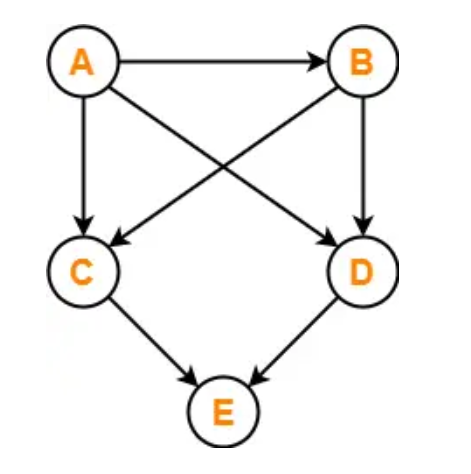A topological sort of this graph would be: A, B, D, C, E. or A, B, C, D, E

It’s important to note that topological sort is only applicable to directed acyclic graphs. If the graph contains cycles, it’s not possible to obtain a valid topological ordering.

Topological sort has various applications, such as task scheduling, dependency resolution, and determining the order of execution in a directed graph.

# Define Minimum Spanning Tree

A Minimum Spanning Tree (MST) is a subset of the edges of a connected, weighted graph that connects all the vertices with the minimum possible total edge weight. In simpler terms, it is a tree that spans all the vertices of the graph with the least total edge weight.

Key properties of a Minimum Spanning Tree:

1. It is a tree: An MST is a connected acyclic graph that does not contain any cycles. It connects all the vertices without forming loops.
2. It spans all vertices: An MST includes all the vertices of the original graph, ensuring that every vertex is reachable from any other vertex through the tree’s edges.
3. It has minimum total weight: The total weight of an MST is the sum of the weights of its edges. The goal of finding an MST is to minimize this total weight, making it the smallest possible among all possible spanning trees of the graph.

Minimum Spanning Tree algorithms, such as Kruskal’s algorithm and Prim’s algorithm, are used to find an MST in a given graph. These algorithms iteratively add edges to the MST while ensuring that the tree remains connected and acyclic.

Applications of Minimum Spanning Tree:

1. Network design: MSTs are used to design efficient and cost-effective network infrastructures, such as laying cables, constructing highways, or connecting cities with minimum cost.
2. Cluster analysis: MSTs are utilized in clustering algorithms to group related data points or objects together based on their proximity or similarity.
3. Approximation algorithms: MSTs are used as building blocks in approximation algorithms for solving optimization problems, such as the traveling salesman problem and facility location problem.
4. Image segmentation: MSTs have applications in image processing, where they can be used to segment images into coherent regions based on pixel similarity.

By finding the minimum spanning tree of a graph, we can identify the subset of edges that form the most efficient and cost-effective way to connect all the vertices.

# Describe Kruskal’s Algorithm

Kruskal’s algorithm is a greedy algorithm used to find a minimum spanning tree (MST) in a connected, weighted graph. It starts with an empty set of edges and gradually adds edges to form the MST.

The algorithm works as follows:

1. Create a set of all the edges in the graph.
2. Sort the edges in non-decreasing order of their weights.
3. Initialize an empty set to store the MST.
4. Iterate through the sorted edges in increasing order of weights.
5. For each edge, check if adding it to the MST creates a cycle. If not, add the edge to the MST.
• To check for cycles, we can use a disjoint set data structure or union-find algorithm.
• If the endpoints of the edge belong to different disjoint sets, add the edge to the MST and merge the sets.
• If the endpoints belong to the same set, skip the edge to avoid creating a cycle.
6. Continue this process until all vertices are included in the MST or until there are no more edges to consider.

OR

MST- KRUSKAL (G, w)

1. A ← ∅

2. for each vertex v ∈ V [G]

3. do MAKE – SET (v)

4. sort the edges of E into non decreasing order by weight w

5. for each edge (u, v) ∈ E, taken in non decreasing order by weight

6. do if FIND-SET (μ) ≠ if FIND-SET (v)

7. then A ← A ∪ {(u, v)}

8. UNION (u, v)

9. return A

Once the algorithm finishes, the resulting set of edges will form the minimum spanning tree of the graph.

# Find Minimum Spanning Tree for the given Graph using Kruskal’s Algorithm

Example: Find the Minimum Spanning Tree of the following graph using Kruskal’s algorithm.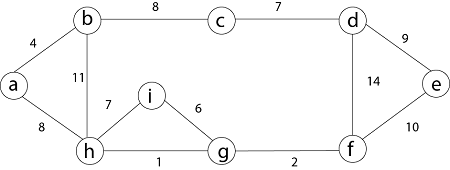First we initialize the set A to the empty set and create |v| trees, one containing each vertex with MAKE-SET procedure. Then sort the edges in E into order by non-decreasing weight.

There are 9 vertices and 12 edges. So MST formed (9-1) = 8 edges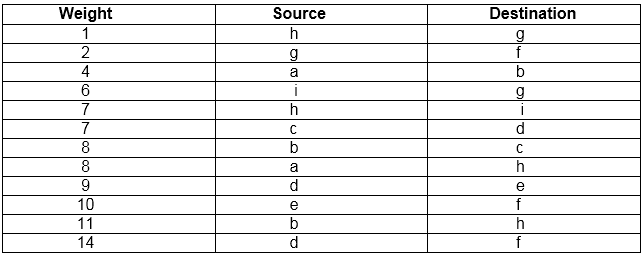Now, check for each edge (u, v) whether the endpoints u and v belong to the same tree. If they do then the edge (u, v) cannot be supplementary. Otherwise, the two vertices belong to different trees, and the edge (u, v) is added to A, and the vertices in two trees are merged in by union procedure.

Step1: So, first take (h, g) edge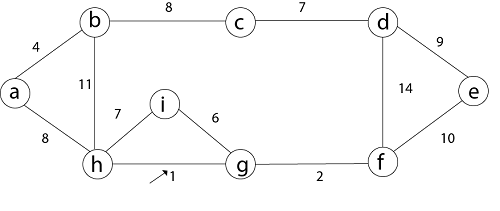Step 2: then (g, f) edge.Step 3: then (a, b) and (i, g) edges are considered, and the forest becomes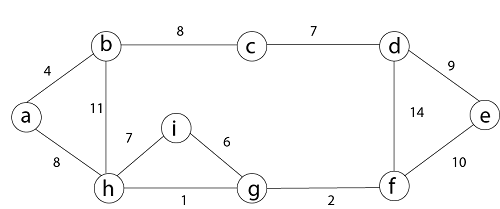Step 4: Now, edge (h, i). Both h and i vertices are in the same set. Thus it creates a cycle. So this edge is discarded.

Then edge (c, d), (b, c), (a, h), (d, e), (e, f) are considered, and the forest becomes.Step 5: In (e, f) edge both endpoints e and f exist in the same tree so discarded this edge. Then (b, h) edge, it also creates a cycle.

Step 6: After that edge (d, f) and the final spanning tree is shown as in dark lines.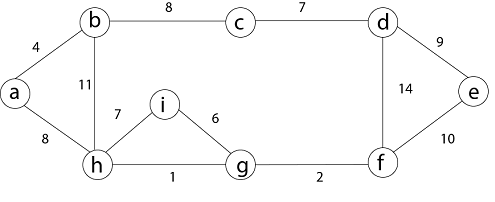Step 7: This step will be required Minimum Spanning Tree because it contains all the 9 vertices and (9 – 1) = 8 edges

1. e → f, b → h, d → f [cycle will be formed]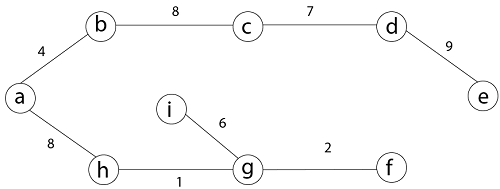This is the Minimum Cost MST

# Describe Prim’s Algorithm

Prim’s algorithm is a greedy algorithm used to find a minimum spanning tree (MST) in a connected, weighted graph. Similar to Kruskal’s algorithm, Prim’s algorithm starts with a single vertex and gradually expands the MST by adding edges that connect the tree to the remaining vertices.

The algorithm works as follows:

1. Choose an arbitrary vertex as the starting point.
2. Create a set to store the MST, initially containing only the starting vertex.
3. Create a priority queue (min-heap) to store the edges and their corresponding weights.
4. Assign an initial weight of infinity to all vertices except the starting vertex, which is assigned a weight of 0.
5. For each adjacent vertex to the starting vertex, insert the corresponding edge and weight into the priority queue.
6. Repeat the following steps until all vertices are included in the MST:

a. Remove the edge with the minimum weight from the priority queue.

b. If the removed edge connects a vertex that is not yet in the MST to a vertex that is already in the MST, add the edge to the MST and include the new vertex.

c. Update the weights of the adjacent vertices of the new vertex if the new edge has a smaller weight than the previous weight.

d. Insert any updated edges into the priority queue.

1. Once all vertices are included in the MST, the algorithm terminates, and the resulting set of edges forms the minimum spanning tree.

Prim’s algorithm guarantees that the resulting tree will be a minimum spanning tree. The algorithm selects the edge with the minimum weight at each step, ensuring that the total weight of the MST is minimized.

Prim’s algorithm, being a minimum spanning tree (MST) algorithm, has various applications in different domains. Some of the key applications include:

1. Network Design: Prim’s algorithm can be used to design efficient and cost-effective network infrastructures, such as laying cables, constructing highways, or connecting cities. It helps in determining the optimal connections that minimize the total cost while ensuring connectivity.
2. Telecommunications: Prim’s algorithm is applied in telecommunications to establish optimal connections between telephone exchanges, cell towers, or network nodes. It helps in minimizing the overall cost of communication infrastructure while maintaining connectivity.
3. Image Segmentation: Prim’s algorithm has applications in image processing, particularly in image segmentation. It can be used to partition an image into regions based on similarity or connectivity, allowing for efficient processing and analysis of specific areas of interest.
4. Clustering: Prim’s algorithm can be employed in clustering algorithms to group similar data points or objects together. It helps in identifying cohesive clusters by establishing connections based on similarity measures.
5. Transportation Planning: Prim’s algorithm can be utilized in transportation planning to optimize routes and connections between different locations. It assists in determining the most efficient and cost-effective paths while considering factors such as distance, traffic, or transportation costs.
6. Facility Location: Prim’s algorithm finds applications in facility location problems, where the objective is to identify optimal locations for establishing facilities (e.g., warehouses, factories) to serve a given set of demand points. It helps in minimizing the total cost of transportation or service provision by determining the best facility locations.
7. Circuit Design: Prim’s algorithm can be used in designing electrical circuits, where the objective is to connect various components while minimizing the overall wire length or the total cost of connections.
8. Data Centers and Cloud Computing: Prim’s algorithm can aid in the optimal placement of data centers or cloud computing infrastructure. It assists in selecting the best locations for data centers or cloud nodes to ensure efficient data processing and minimize communication costs.

Overall, Prim’s algorithm finds applications in various domains that involve network optimization, infrastructure planning, data analysis, and clustering problems. Its ability to find a minimum spanning tree helps in solving optimization problems where the objective is to minimize costs or establish efficient connections between entities.

# Find Minimal Spanning Tree for a given Graph using Prim’s Algorithm

Example: Consider a weighted graph given below and find the MST using Prim’s algorithm.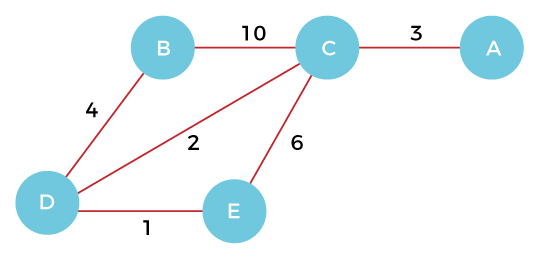Step 1 – First, we have to choose a vertex from the above graph. Let’s choose B.Step 2 – Now, we have to choose and add the shortest edge from vertex B. There are two edges from vertex B that are B to C with weight 10 and edge B to D with weight 4. Among the edges, the edge BD has the minimum weight. So, add it to the MST.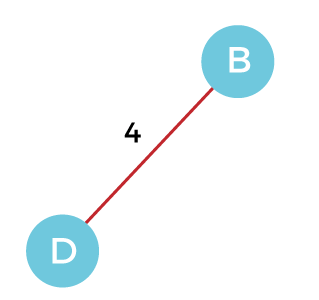Step 3 – Now, again, choose the edge with the minimum weight among all the other edges. In this case, the edges DE and CD are such edges. Add them to MST and explore the adjacent of C, i.e., E and A. So, select the edge DE and add it to the MST.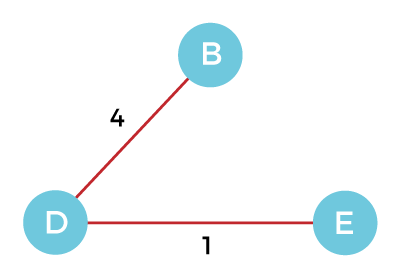Step 4 – Now, select the edge CD, and add it to the MST.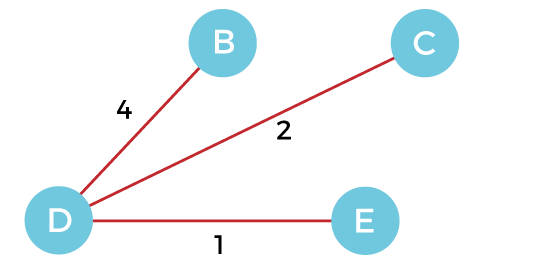Step 5 – Now, choose the edge CA. Here, we cannot select the edge CE as it would create a cycle to the graph. So, choose the edge CA and add it to the MST.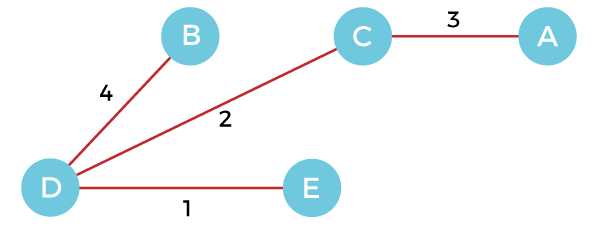So, the graph produced in step 5 is the minimum spanning tree of the given graph. The cost of the MST is given below

Cost of MST = 4 + 2 + 1 + 3 = 10 units.

# Define the following terms: Transitive Closure, Activity Network, and Shortest Path

1. Transitive Closure: In graph theory, the transitive closure of a directed graph is a graph that represents the reachability relationship between vertices. It determines all possible paths between vertices, including indirect paths through intermediate vertices. The transitive closure of a graph G is denoted as TC(G) and is obtained by adding edges to G to connect all pairs of vertices that have a path between them. It is often represented as a binary matrix, where the entry TC[i][j] is 1 if there is a path from vertex i to vertex j, and 0 otherwise.
2. Activity Network: An activity network, also known as a precedence network or a project network, is a graphical representation of a project or a set of activities and their dependencies. It is commonly used in project management to visualize the sequence and relationships between tasks or activities required to complete a project. The network consists of nodes representing activities and directed edges representing the precedence relationships or dependencies between activities. Activity networks can be represented using different notations, such as arrow diagrams (also known as arrow networks or activity-on-arrow networks) or node diagrams (also known as node networks or activity-on-node networks).
3. Shortest Path: In graph theory, the shortest path refers to the path between two vertices in a graph that has the minimum total weight or cost. The weight or cost of a path is determined by the sum of the weights or costs of its constituent edges. The concept of shortest path is commonly used in various applications, such as routing algorithms in computer networks, GPS navigation systems, transportation planning, and optimization problems. There are different algorithms to find the shortest path in a graph, including Dijkstra’s algorithm, Bellman-Ford algorithm, and Floyd-Warshall algorithm, each with its own characteristics and requirements.

# Discuss Warshall’s Algorithm

Warshall’s algorithm, also known as the Floyd-Warshall algorithm, is a dynamic programming algorithm used to find the shortest path between all pairs of vertices in a weighted directed graph. It efficiently computes the transitive closure of the graph and solves the All-Pairs Shortest Path (APSP) problem.

The algorithm works as follows:

1. Initialize a matrix, often denoted as D, to represent the distances between vertices. Each entry D[i][j] in the matrix initially represents the weight of the edge between vertices i and j, or infinity if there is no edge.
2. For each vertex k in the graph, iterate through all pairs of vertices i and j. Check if the distance from i to j through k (D[i][k] + D[k][j]) is smaller than the current distance D[i][j]. If it is, update D[i][j] to the new smaller distance.
3. Repeat step 2 for all vertices in the graph. Each iteration of the outer loop considers an additional vertex as an intermediate vertex and updates the distances between pairs of vertices.
4. After all iterations are completed, the matrix D will contain the shortest distances between all pairs of vertices.

Warshall’s algorithm is based on the concept of dynamic programming and the principle of optimality. By considering all possible intermediate vertices, the algorithm systematically updates the distances between vertices to find the shortest path. The algorithm guarantees that at each iteration, the distances are optimized using the available intermediate vertices.

The time complexity of Warshall’s algorithm is O(V^3), where V is the number of vertices in the graph. The space complexity is also O(V^2) since it requires a matrix of size V x V to store the distances.

OR

Warshall(A[1…n, 1…n]) // A is the adjacency matrix

R(0) ← A

for k ← 1 to n do

for i ← 1 to n do

for j ← to n do

R(k)[i, j] ← R(k-1)[i, j] or (R(k-1)[i, k] and R(k-1)[k, j])

return R(n)

The Floyd-Warshall algorithm is versatile and can handle graphs with both positive and negative edge weights, as long as the graph does not contain negative cycles. However, it is not suitable for large graphs due to its cubic time complexity. For graphs with relatively small sizes or dense graphs where the number of edges is close to the maximum possible, Warshall’s algorithm provides an efficient solution to find the shortest paths between all pairs of vertices.

Here’s an example of a C implementation of the Floyd-Warshall algorithm:

#include <stdio.h>

#include <limits.h>

#define INF INT_MAX

#define V 4

void floydWarshall(int graph[V][V]) {

int dist[V][V];

int i, j, k;

// Initialize the distance matrix with the graph values

for (i = 0; i < V; i++) {

for (j = 0; j < V; j++) {

dist[i][j] = graph[i][j];

}

}

// Applying the Floyd-Warshall algorithm

for (k = 0; k < V; k++) {

for (i = 0; i < V; i++) {

for (j = 0; j < V; j++) {

// Check if there’s a shorter path through vertex k

if (dist[i][k] != INF && dist[k][j] != INF && dist[i][k] + dist[k][j] < dist[i][j]) {

dist[i][j] = dist[i][k] + dist[k][j];

}

}

}

}

// Printing the shortest distances

for (i = 0; i < V; i++) {

for (j = 0; j < V; j++) {

if (dist[i][j] == INF) {

printf(“INF\t”);

} else {

printf(“%d\t”, dist[i][j]);

}

}

printf(“\n”);

}

}

int main() {

int graph[V][V] = {{0, 5, INF, 10},

{INF, 0, 3, INF},

{INF, INF, 0, 1},

{INF, INF, INF, 0}};

floydWarshall(graph);

return 0;

}

In this program, we have a graph represented as a 2D matrix, where V is the number of vertices. The INF constant represents infinity. The floydWarshall function takes the graph as input and applies the Floyd-Warshall algorithm to compute the shortest distances between all pairs of vertices. Finally, the program prints the shortest distances between all pairs of vertices.

Make sure to include the necessary headers (stdio.h, limits.h) and adjust the graph size (V) and the values in the graph matrix according to your specific graph.

# Find All Pair Shortest Paths for a given Graph using Warshall’s Algorithm

Example: Consider the following graph and apply Warshall’s Algorithm.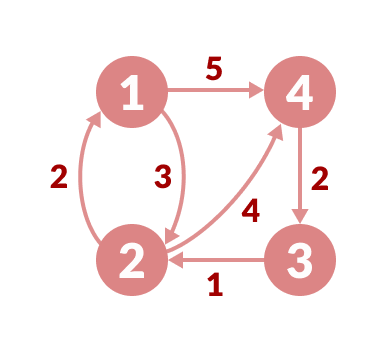Follow the steps below to find the shortest path between all the pairs of vertices.

1. Create a matrix A0 of dimension n*n where n is the number of vertices. The row and the column are indexed as i and j respectively. i and j are the vertices of the graph. Each cell A[i][j] is filled with the distance from the ith vertex to the jth vertex. If there is no path from ith vertex to jth vertex, the cell is left as infinity.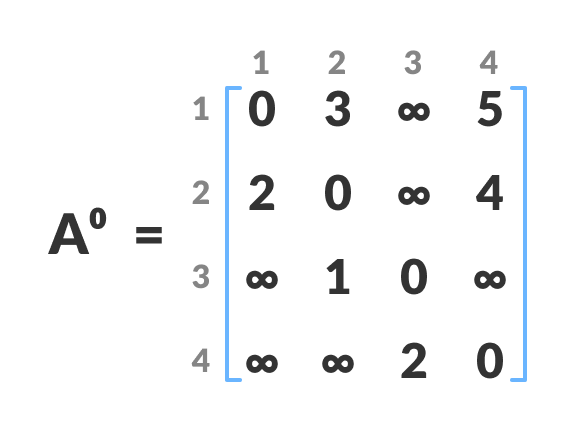Fill each cell with the distance between ith and jth vertex

1. Now, create a matrix A1 using matrix A0. The elements in the first column and the first row are left as they are. The remaining cells are filled in the following way.Let k be the intermediate vertex in the shortest path from source to destination. In this step, k is the first vertex. A[i][j] is filled with

(A[i][k] + A[k][j]) if (A[i][j] > A[i][k] + A[k][j]).

That is, if the direct distance from the source to the destination is greater than the path through the vertex k, then the cell is filled with A[i][k] + A[k][j].

In this step, k is vertex 1. We calculate the distance from source vertex to destination vertex through this vertex k.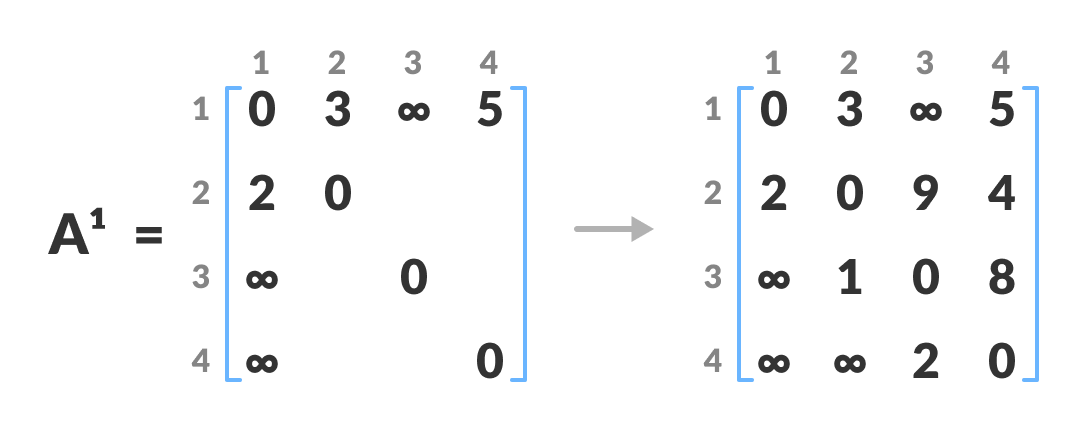Calculate the distance from the source vertex to destination vertex through this vertex k
For example: For A1[2, 4], the direct distance from vertex 2 to 4 is 4 and the sum of the distance from vertex 2 to 4 through vertex (ie. from vertex 2 to 1 and from vertex 1 to 4) is 7. Since 4 < 7, A0[2, 4] is filled with 4.

1. Similarly, A2 is created using A1. The elements in the second column and the second row are left as they are.In this step, k is the second vertex (i.e. vertex 2). The remaining steps are the same as in step 2.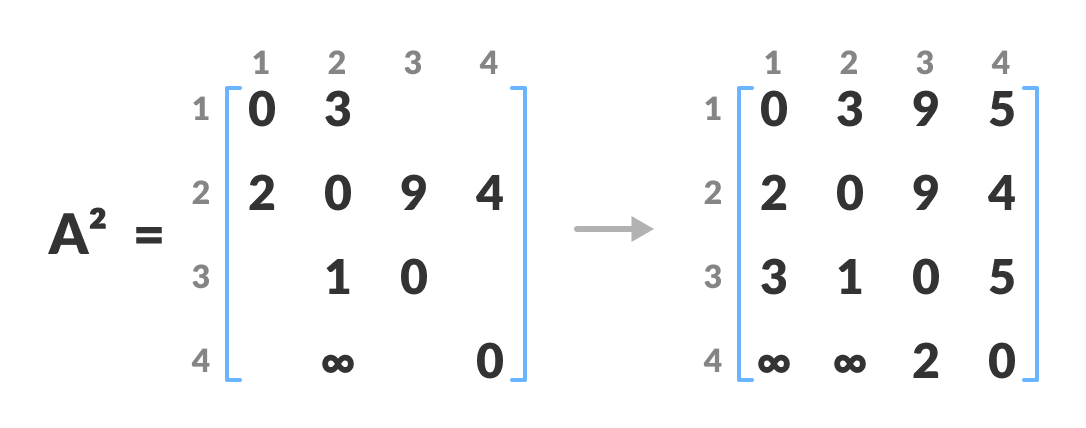Calculate the distance from the source vertex to destination vertex through this vertex 2

1. Similarly, A3 and A4 is also created.
2. Calculate the distance from the source vertex to destination vertex through this vertex 3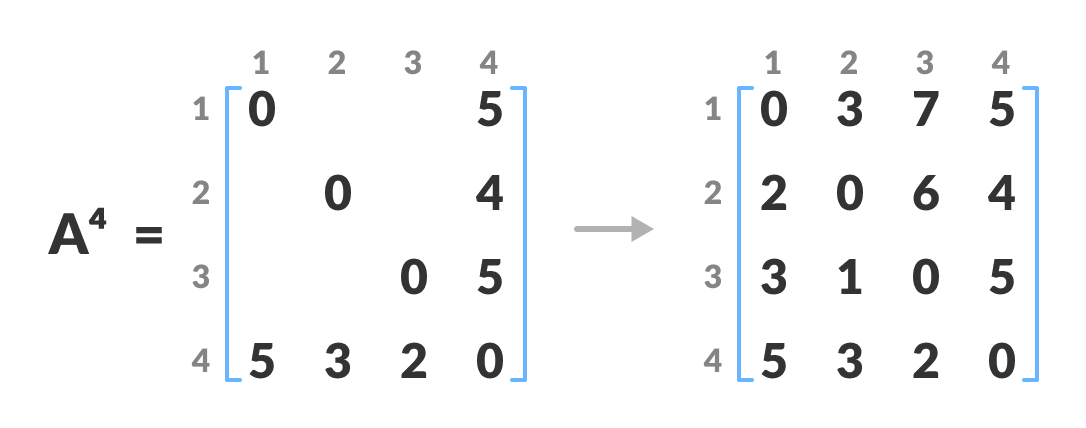Calculate the distance from the source vertex to destination vertex through this vertex 4

1. A4 gives the shortest path between each pair of vertices.

# Describe Dijkstra’s Algorithm

Dijkstra’s algorithm is a popular graph traversal algorithm used to find the shortest path from a source vertex to all other vertices in a weighted directed graph. It works on graphs with non-negative edge weights and produces the shortest path tree.

The algorithm operates as follows:

1. Initialize the distance from the source vertex to all other vertices as infinity, except for the source vertex itself, which is set to 0. Maintain a priority queue (often implemented as a min-heap) to store vertices and their corresponding distances from the source.
2. Select the vertex with the smallest distance from the priority queue. This vertex is typically the one with the minimum distance among the vertices that have not yet been processed.
3. For the selected vertex, consider all its neighboring vertices. Calculate the distance from the source vertex to each neighboring vertex by adding the weight of the edge connecting them to the distance of the selected vertex. If this distance is smaller than the current distance stored for the neighboring vertex, update the distance.
4. After updating the distances, if any, reposition the vertices in the priority queue based on their updated distances.
5. Repeat steps 2 to 4 until all vertices have been processed or the priority queue becomes empty.
6. The resulting distances stored for each vertex represent the shortest path distance from the source vertex to all other vertices in the graph.
7. To obtain the actual shortest path from the source vertex to a specific destination vertex, backtrack from the destination vertex using the recorded distances and select the edges with corresponding minimum distances until the source vertex is reached.

Dijkstra’s algorithm guarantees that once a vertex is processed, its final shortest path distance from the source is determined. It explores vertices in increasing order of their distances from the source, gradually expanding the shortest path tree until all vertices have been visited.

The time complexity of Dijkstra’s algorithm is typically O((V + E) log V), where V is the number of vertices and E is the number of edges in the graph. This complexity arises due to the operations performed on the priority queue.

Dijkstra’s algorithm is widely used in various applications, including route planning, network routing protocols, and resource allocation in computer networks, among others.

Here’s an implementation of Dijkstra’s algorithm in C:

#include <stdio.h>

#include <stdbool.h>

#include <limits.h>

#define V 6 // Number of vertices in the graph

int findMinDistance(int dist[], bool visited[]) {

int min = INT_MAX, min_index;

for (int v = 0; v < V; v++) {

if (!visited[v] && dist[v] <= min) {

min = dist[v];

min_index = v;

}

}

return min_index;

}

void printSolution(int dist[]) {

printf(“Vertex\tDistance from Source\n”);

for (int i = 0; i < V; i++) {

printf(“%d\t%d\n”, i, dist[i]);

}

}

void dijkstra(int graph[V][V], int source) {

int dist[V]; // Array to store the shortest distance from source to each vertex

bool visited[V]; // Array to keep track of visited vertices

// Initialize dist[] and visited[]

for (int i = 0; i < V; i++) {

dist[i] = INT_MAX;

visited[i] = false;

}

dist[source] = 0; // Distance from source to itself is always 0

// Find shortest path for all vertices

for (int count = 0; count < V – 1; count++) {

int u = findMinDistance(dist, visited);

visited[u] = true;

// Update distances of adjacent vertices of the selected vertex

for (int v = 0; v < V; v++) {

if (!visited[v] && graph[u][v] && dist[u] != INT_MAX && dist[u] + graph[u][v] < dist[v]) {

dist[v] = dist[u] + graph[u][v];

}

}

}

printSolution(dist); // Print the shortest distances

}

int main() {

int graph[V][V] = {{0, 5, 10, 0, 0, 0},

{0, 0, 2, 1, 0, 0},

{0, 0, 0, 0, 4, 0},

{0, 0, 0, 0, 0, 3},

{0, 0, 0, 2, 0, 6},

{0, 0, 0, 0, 0, 0}};

int source = 0; // Source vertex

dijkstra(graph, source);

return 0;

}

In this program, we have a graph represented as a 2D matrix graph with the number of vertices V. The dijkstra function implements the Dijkstra’s algorithm, taking the graph and the source vertex as inputs. It initializes the distance array dist and the visited array visited, and iteratively finds the shortest distances from the source to all other vertices. The findMinDistance function is a helper function used to find the vertex with the minimum distance that has not been visited yet. Finally, the printSolution function is used to print the shortest distances from the source to all vertices.

Make sure to adjust the size of the graph (V) and the values in the graph matrix according to your specific graph.

Applications:

Dijkstra’s algorithm has numerous applications in various domains. Some of the common applications include:

1. Shortest Path Routing: Dijkstra’s algorithm is extensively used in computer networks and routing protocols to determine the shortest path between two network nodes. It helps in efficient packet forwarding and optimizing network performance.
2. GPS Navigation: Navigation systems employ Dijkstra’s algorithm to calculate the shortest routes between two locations. It assists in providing real-time directions, optimizing travel time, and avoiding traffic congestion.
3. Flight Path Planning: Dijkstra’s algorithm is employed in the airline industry to determine the most efficient flight routes between airports. It helps in minimizing fuel consumption, reducing travel time, and optimizing flight schedules.
4. Robot Path Planning: Dijkstra’s algorithm is utilized in robotics for path planning of autonomous robots. It enables robots to navigate through obstacles while finding the shortest path to reach a specific destination.
5. Network Analysis: Dijkstra’s algorithm is employed in network analysis to determine the critical path in project management. It helps in identifying the sequence of tasks with the least amount of time required to complete a project.
6. Image Segmentation: Dijkstra’s algorithm is used in image processing for segmentation, where it assists in partitioning an image into regions based on similarities in pixel intensities. It helps in object recognition, image understanding, and computer vision applications.
7. DNA Sequencing: In bioinformatics, Dijkstra’s algorithm is used for DNA sequencing alignment, which involves finding the most similar sequences between DNA samples. It aids in identifying genetic variations, studying gene functions, and understanding evolutionary relationships.
8. Social Network Analysis: Dijkstra’s algorithm is utilized in social network analysis to measure the closeness centrality of individuals within a network. It helps in identifying influential individuals and understanding information flow dynamics in social networks.

These are just a few examples of the applications of Dijkstra’s algorithm. Its versatility and efficiency make it a fundamental tool in various fields where finding the shortest path or optimizing network connectivity is crucial.

# Find the Single Source Shortest Path for the given Graph Dijkstra’s Algorithm

Let’s consider an example to illustrate the application of Dijkstra’s algorithm in finding the shortest path in a network.

Suppose we have the following network of cities with their respective distances:

City A –> City B: 5 units

City A –> City C: 2 units

City B –> City D: 3 units

City B –> City E: 4 units

City C –> City D: 1 unit

City D –> City E: 2 units

Our goal is to find the shortest path from City A to City E using Dijkstra’s algorithm.

Step 1: Initialize the algorithm

• Set the source vertex as City A and assign a distance of 0 to it.
• Assign a distance of infinity to all other vertices: City B, City C, City D, and City E.

Step 2: Explore the neighbors and update distances

• Start from City A and consider its neighbors: City B and City C.
• Update the distances of City B and City C as follows:
• Distance of City B: 5 units (from A to B)
• Distance of City C: 2 units (from A to C)

Step 3: Select the vertex with the smallest distance

• Among the remaining vertices, select the one with the smallest distance. In this case, it’s City C with a distance of 2 units.

Step 4: Explore the neighbors of the selected vertex and update distances

• Consider the neighbors of City C: City D.
• Update the distance of City D as follows:
• Distance of City D: 3 units (from A to C to D)

Step 5: Select the next vertex with the smallest distance

• Among the remaining vertices, select the one with the smallest distance. In this case, it’s City D with a distance of 3 units.

Step 6: Explore the neighbors of the selected vertex and update distances

• Consider the neighbors of City D: City B and City E.
• Update the distances of City B and City E as follows:
• Distance of City B: 3 units (from A to C to D to B)
• Distance of City E: 5 units (from A to C to D to E)

Step 7: Select the next vertex with the smallest distance

• Among the remaining vertices, select the one with the smallest distance. In this case, it’s City B with a distance of 3 units.

Step 8: Explore the neighbors of the selected vertex and update distances

• Consider the neighbors of City B: City D and City E.
• Since the distances of City D and City E are already updated, there is no change.

Step 9: Select the next vertex with the smallest distance

• Among the remaining vertices, select the one with the smallest distance. In this case, it’s City E with a distance of 5 units.

Step 10: Final result

• After considering all the vertices, the shortest distance from City A to City E is 5 units.

The shortest path from City A to City E is A -> C -> D -> E with a total distance of 5 units.

This example demonstrates how Dijkstra’s algorithm is used to find the shortest path in a network by considering the distances between vertices and iteratively updating the distances until the shortest path is determined.